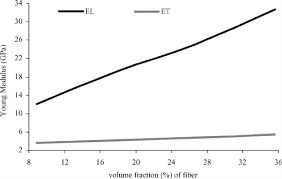## How to Calculate and Solve for Elastic Modulus on Longitudinal Direction | Composites

The elastic modulus on longitudinal direction is illustrated by the image below.To compute for elastic modulus on longitudinal direction, three essential parameters are needed and these parameters are Elastic Modulus of the Matrix (Em), Elastic Modulus of the Fibre (Ef) and Volume Fraction of the Fibre (Vf).

The formula for calculating elastic modulus on longitudinal direction:

Eu = Em(1 – Vf) + EfVf

Where:

Eu = Elastic Modulus on Longitudinal Direction
Em = Elastic Modulus of the Matrix
Ef = Elastic Modulus of the Fibre
Vf = Volume Fraction of the Fibre

Let’s solve an example;
Find the elastic modulus on longitudinal direction when the elastic modulus of the matrix is 12, the elastic modulus of the fibre is 10 and volume fraction of the fibre is 4.

This implies that;

Em = Elastic Modulus of the Matrix = 12
Ef = Elastic Modulus of the Fibre = 10
Vf = Volume Fraction of the Fibre = 4

Eu = Em(1 – Vf) + EfVf
Eu = (12)(1 – 4) + (10)(4)
Eu = (12)(-3) + (40)
Eu = (-36) + (40)
Eu = 4

Therefore, the elastic modulus on longitudinal direction is 4 Pa.